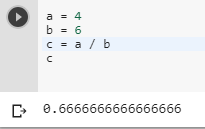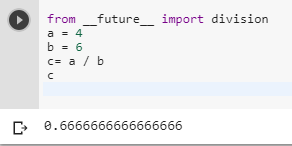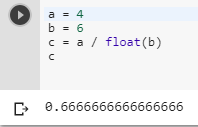2 views
in Python

I have two integer values a and b, but I need their ratio in floating point. I know that a < b, and I want to calculate a / b, so if I use integer division I'll always get 0 with a remainder of a.

How can I force c to be a floating point number in Python in the following?

c= a / b

by (106k points)
• Python 3 gives us the feature that we can directly get the value as a floating point by using division (/) operator.• For getting the value in a floating point you can import a library called __future__.

from __future__ import division

a = 4

b = 6

c= a / b

CHere is the way by which you can get your desired output.

• Another thing you can do is type-cast the b value into a float. That will also give you float value as output.